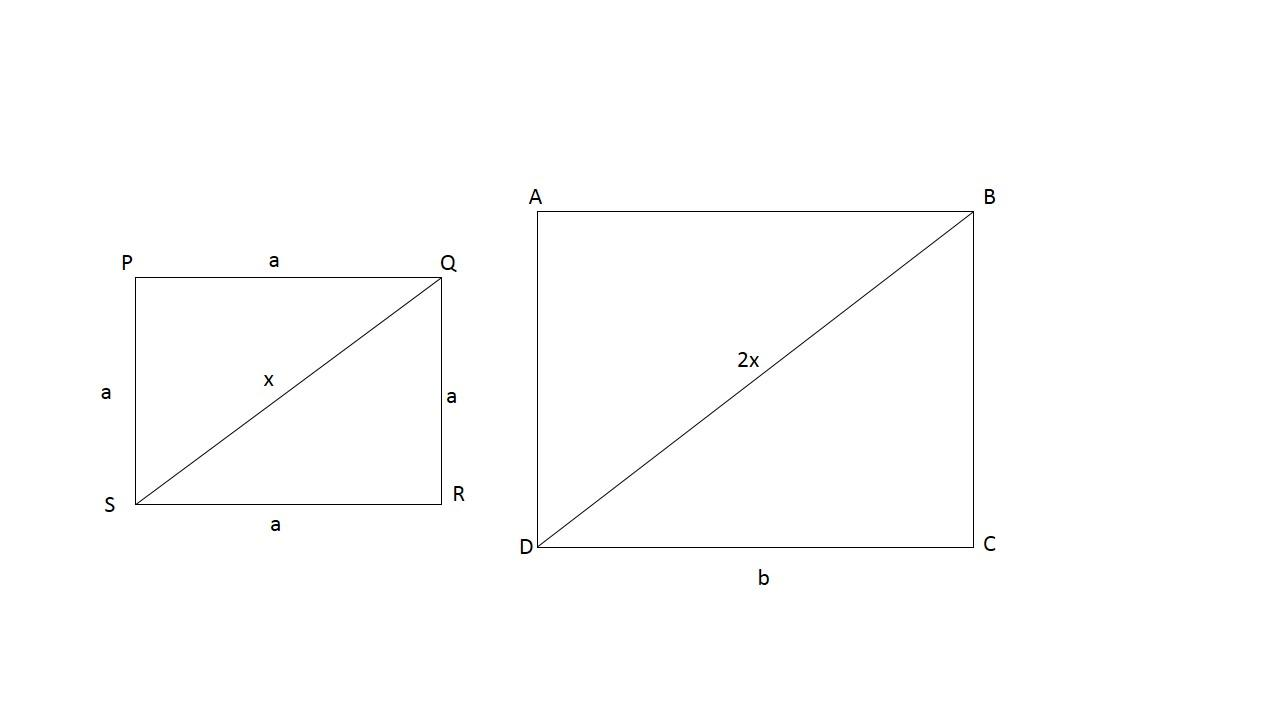QuestionAnswers

# If a diagonal of a square is doubled, then the area of the square becomes m times. Find m.

Hint: Use Pythagoras theorem to find the diagonal of the square, then double it to get the new length. Use this length to compute the area of the resultant square.

Consider a square of side length ‘a’Using Pythagoras theorem on $\Delta \,\text{QRS}$,
\begin{align} & \text{S}{{\text{R}}^{\text{2}}}\,\text{+}\,\text{Q}{{\text{R}}^{\text{2}}}\,\text{=}\,\text{Q}{{\text{S}}^{\text{2}}} \\ & {{\text{a}}^{\text{2}}}\,\text{+}\,{{\text{a}}^{\text{2}}}\,\text{=}\,\text{Q}{{\text{S}}^{\text{2}}} \end{align}
$\therefore$ length of diagonal $\text{QS}\,\text{=}\,\sqrt{\text{2}}\text{a}$
Now, according to the question, the diagonal of square
\begin{align} & \text{ABCD}\,\text{=}\,\text{2 }\!\!\times\!\!\text{ }\,\text{diagonal}\,\text{of PQRS} \\ & \text{=}\,\text{2}\,\times \,\sqrt{2}a \\ & =\,2\sqrt{2}a. \end{align}
Let us apply the Pythagoras theorem again on $\Delta \,\text{BCD}$
$\text{C}{{\text{D}}^{\text{2}}}\,\text{+}\,\text{B}{{\text{C}}^{\text{2}}}\,\text{=}\,\text{BD}$
But $\text{BD}\,\text{=}\,\text{2}\sqrt{2}\text{a}$, as derived before
\begin{align} & \therefore \,\text{C}{{\text{D}}^{\text{2}}}\,\text{+}\,\text{B}{{\text{C}}^{\text{2}}}\,\text{=}\,\text{8}{{\text{a}}^{\text{2}}} \\ & \text{But}\,\text{CD}\,\text{=}\,\text{BC}\,\text{=}\,\text{b}\,\text{(New square side)} \\ & \text{2}{{\text{b}}^{2}}\,=\,8{{\text{a}}^{2}} \\ & \text{b}\,\text{=}\,\text{2a} \end{align}
So the new square has a side that’s double the length of the old square.
Let’s finally compare areas
\begin{align} & \text{Area}\,\text{of}\,\text{PQRS}\,\text{=}\,{{\text{a}}^{\text{2}}} \\ & \text{Area}\,\text{of}\,\text{ABCD}\,\text{=}\,{{\text{b}}^{\text{2}}}\,\text{=}\,{{\text{(2a)}}^{\text{2}}}\,\text{=}\,\text{4}{{\text{a}}^{\text{2}}} \\ \end{align}
$\therefore \,\dfrac{\text{Area}\,\text{of}\,\text{ABCD}}{\text{Area}\,\text{of}\,\text{PQRS}}\,\text{=}\,\dfrac{\text{4}{{\text{a}}^{\text{2}}}}{{{\text{a}}^{\text{2}}}}\,\text{=}\,\text{4}$
Hence m = 4

Note: Remember that if a square changes its length by la times, its area changes by a factor of ${{\text{l}}^{2}}$, depending on whether the length is increase or decreases.
\begin{align} & \text{If}\,\text{a}\,\to \,\text{la}\,\text{then}\,\text{area}\,\to \,{{\text{l}}^{\text{2}}}\,\text{area} \\ & \text{If}\,\text{a}\,\to \,\dfrac{\text{l}}{\text{l}}\text{a}\,\text{then}\,\text{area}\,\to \,\dfrac{\text{l}}{{{\text{l}}^{\text{2}}}}\,\text{area} \\ \end{align}